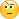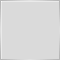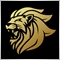# What is the value of one pixel?581

What is the value of one pixel on the Y axis of the current chart?

I need this value so that I can calculate the value of 600 pixels.

This is because I want 600 pixel take profit level, and not 600 Points or 600 pips.

I want a uniform take profit levels on all charts, regardless of their Point values or Pip values.

It is time to trade in terms of pixels. Thank you in advance somebody.38701

macpee:
What is the value of one pixel on the Y axis of the current chart? Thank you in advance.

The value ?A pixel doesn't have a "value".

What do you mean ?31030

macpee:
What is the value of one pixel on the Y axis of the current chart? Thank you in advance.
I think it is not even in Forex
It is in photoshop ;)

Maybe you are sending to another website581

Alain Verleyen:

The value ? A pixel doesn't have a "value".

What do you mean ?

If a pixel does not have a value, how then is it possible to draw a line using x and y axes (of course these variables take values in pixels) or to create buttons etc that use the coordinates?Moderator
2308

double max_price  = ChartGetDouble(0,CHART_PRICE_MAX),
min_price  = ChartGetDouble(0,CHART_PRICE_MIN);
long chart_height = ChartGetInteger(0,CHART_HEIGHT_IN_PIXELS);
printf("1 pixel = %f",(max_price-min_price)/chart_height);581

honest_knave:
double max_price  = ChartGetDouble(0,CHART_PRICE_MAX),
min_price  = ChartGetDouble(0,CHART_PRICE_MIN);
long chart_height = ChartGetInteger(0,CHART_HEIGHT_IN_PIXELS);
printf("1 pixel = %f",(max_price-min_price)/chart_height);
Thanks a lot. I think this is a better reply which I have to try to use.Moderator
12729

macpee:
If a pixel does not have a value, how then is it possible to draw a line using x and y axes (of course these variables take values in pixels) or to create buttons etc that use the coordinates?Documentation on MQL5: Chart Operations / ChartXYToTimePrice
• www.mql5.com
Chart Operations / ChartXYToTimePrice - Reference on algorithmic/automated trading language for MetaTrader 5Moderator
2308

macpee:
If a pixel does not have a value, how then is it possible to draw a line using x and y axes (of course these variables take values in pixels) or to create buttons etc that use the coordinates?

It is probably worth mentioning that there are 2 different ways to draw objects.

• Using time / price... such as OBJ_TREND and OBJ_TEXT
• These objects will move along the screen as you scroll the chart left/right/up/down.
• Using X/Y pixels... such as OBJ_BUTTON and OBJ_LABEL
• These objects will not move on the screen, even if the chart scrolls.

Sometimes it is necessary to mix these up e.g. you want to draw an object relative to time on the X axis, and pixels on the Y axis.

Under these circumstances, you will want to use ChartXYToTimePrice() or ChartTimePriceToXY() as Marco has suggested.581

Marco vd Heijden:
Wow! I think this one should be a lot useful. Thanks a lot. I will combine it with the following reverse function:

bool  ChartTimePriceToXY(
long           chart_id,     // Chart ID
int            sub_window,   // The number of the subwindow
datetime       time,         // Time on the chart
double         price,        // Price on the chart
int&           x,            // The X coordinate for the time on the chart
int&           y             // The Y coordinates for the price on the chart
);Moderator
20477

Trying to calculate TP, SL etc using pixels would likely be unreliable. Zooming in or out would affect the calculations. What about a high def screen with more than the standard DPI?

There could be a very large bar at the far left of the screen, when that bar moves out of the visible area then any values calculated would cgange again as the chart adjusts to the total range.

What about when you have the terminal  and/or strategy tester windows open?

There would be nothing uniform at all by using pixels160304

macpee:
Wow! I think this one should be a lot useful. Thanks a lot. I will combine it with the following reverse function:

bool  ChartTimePriceToXY(
long           chart_id,     // Chart ID
int            sub_window,   // The number of the subwindow
datetime       time,         // Time on the chart
double         price,        // Price on the chart
int&           x,            // The X coordinate for the time on the chart
int&           y             // The Y coordinates for the price on the chart
);
According to that logic, what is the price at pixel coordinate 0,0? And why is it not 0? And why is it changing as you scroll the data in the terminal? And why does it change when you change the scale of the displayed chart or you change the chart zoom? And why is it changed at same pixels when a new bar is formed? And why is it different on same pixel coordinates on your terminal from my terminal? Use prices where prices are available, unless you plan to trade pixels